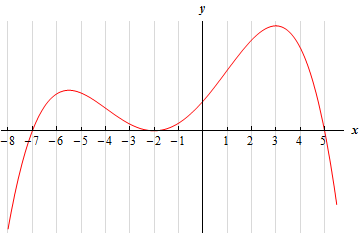Paul's Online Notes
Home / Calculus I / Applications of Derivatives / The Shape of a Graph, Part I
Show Mobile Notice Show All Notes Hide All Notes
Mobile Notice
You appear to be on a device with a "narrow" screen width (i.e. you are probably on a mobile phone). Due to the nature of the mathematics on this site it is best views in landscape mode. If your device is not in landscape mode many of the equations will run off the side of your device (should be able to scroll to see them) and some of the menu items will be cut off due to the narrow screen width.

### Section 4.5 : The Shape of a Graph, Part I

3. Below is the graph of the derivative of a function. From this graph determine the intervals in which the function increases and decreases.Hint : Be careful with this problem. The graph is of the derivative of the function and so we don’t just write down intervals where the graph is increasing and decreasing. Recall how the derivative tells us where the function is increasing and decreasing and this problem is not too bad.
Show Solution

We have to be careful and not do this problem as we did the first two practice problems. The graph given is the graph of the derivative and not the graph of the function. So, the answer is not just where the graph is increasing or decreasing.

Instead we need to recall that the sign of the derivative tells us where the function is increasing and decreasing. If the derivative is positive (i.e. its graph is above the $$x$$-axis) then the function is increasing and if the derivative is negative (i.e. its graph is below the $$x$$-axis) then the function is decreasing.

So, it is fairly clear where the graph is above/below the $$x$$-axis and so we have the following intervals of increase/decrease.

$\require{bbox} \bbox[2pt,border:1px solid black]{{{\mbox{Increasing : }}\left( { - 7, - 2} \right)\,\,\,\,\& \,\,\,\left( { - 2,5} \right)\hspace{0.25in}\hspace{0.25in}{\mbox{Decreasing : }}\,\,\left( { - \infty , - 7} \right)\,\,\,\,\,\,\& \,\,\,\,\,\left( {5,\infty } \right)}}$